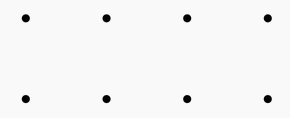###### back to index | new

Let $n$ be a positive integer, prove that in this series $$\Big\lfloor{\frac{n}{1}}\Big\rfloor, \Big\lfloor{\frac{n}{2}}\Big\rfloor, \Big\lfloor{\frac{n}{3}}\Big\rfloor \cdots \Big\lfloor{\frac{n}{n}}\Big\rfloor$$, there are less than $2\sqrt{n}$ integers distinct.

Let $A={1,2,3,4}$, and $f$ and $g$ be randomly chosen (not necessarily distinct) functions from $A$ to $A$. Find the probability that the range of $f$ and the range of $g$ are disjoint.

Let a, b, c, d, and e be distinct integers such that $(6-a)(6-b)(6-c)(6-d)(6-e)=45$ What is $a+b+c+d+e$?

What is the area enclosed by the graph of $|3x|+|4y|=12$?

How many non-congruent triangles have vertices at three of the eight points in the array shown below?Ten chairs are evenly spaced around a round table and numbered clockwise from $1$ through $10$. Five married couples are to sit in the chairs with men and women alternating, and no one is to sit either next to or across from his/her spouse. How many seating arrangements are possible?

Let $\alpha$ and $\beta$ be two real roots of the equation $x^2 + x - 4=0$. Find the value of $\alpha^2 - 5\beta + 10$ without computing the value of $\alpha$ and $\beta$.

A spider has one sock and one shoe for each of its eight legs. In how many different orders can the spider put on its socks and shoes, assuming that, on each leg, the sock must be put on before the shoe.

Let $a$ and $b$ be non-negative real numbers such that $a + b = 2$. Show that: $$\frac{1}{a^2+1}+\frac{1}{b^2 +1} \le \frac{2}{ab+1}$$

Let $X$ be the integer part of $\left(3+\sqrt{7}\right)^n$ where $n$ is a positive integer. Show that $X$ must be odd.

Let $n$ be a positive integer. Show that the smallest integer that is larger than $(1+\sqrt{3})^{2n}$ is divisible by $2^{n+1}$.

Let $m=4k+1$ where $k$ is a non-negative integer. Show that $$a=\binom{n}{1}+m\binom{n}{3}+m^2\binom{n}{5}+\cdots+m^{\frac{n-1}{2}}\binom{n}{n}$$

is divisible by $2^{n-1}$, where $n$ is an odd number.

Let sequence $\{a_n\}$ satisfy $a_0=0, a_1=1$, and $a_n = 2a_{n-1}+a_{n-2}$. Show that $2^k\mid n$ if and only if $2^k\mid a_n$.

Let the integer and decimal part of $(5\sqrt{2}+7)^{2n+1}$ be $I$ and $D$ respectively. Show that $(I+D)D$ is a constant.

Let $n$ be a non-negative integer. Show that $2^{n+1}$ divides the value of $\left\lfloor{(1+\sqrt{3})^{2n+1}}\right\rfloor$ where function $\lfloor{x}\rfloor$ returns the largest integer not exceeding the give real number $x$.

Alan and Barbara play a game in which they take turns filling entries of an initially empty $1024$ by $1024$ array. Alan plays first. At each turn, a player chooses a real number and places it in a vacant entry. The game ends when all the entries are filled. Alan wins if the determinant of the resulting matrix is nonzero; Barbara wins if it is zero. Which player has a winning strategy?

Determine all roots, real or complex, of the following system \begin{align} x+y+z &= 3\\ x^2+y^2+z^2 &= 3\\ x^3+y^3+z^3 &= 3 \end{align}

$\textbf{Color the Grid}$

Two geniuses are playing a game of coloring a $2\times n$ grid where $n$ is an odd integer. Each of them in turn picks a uncolored cell and colors it in either green or red until all the cells are filled. At the end of the game, if the number of adjacent pairs with the same color is greater than the number of adjacent pairs with different colors, then the person who picks and colors first wins the game. (An adjacent pair consists of two cells next to each other.) Otherwise, if there are more adjacent pairs with different colors than those with same color, the person who starts later wins. If these two numbers are the same, the result is a tie. Who will win if both players make no mistake?

$\textbf{Coins on a Table}$

Joe invites you to play a game with him by placing quarters on a rectangular shaped table. Each person places one coin in turn. Coins cannot overlap. The person who cannot find enough space to place the next coin loses the game. Do you want to play first or let Joe play first?